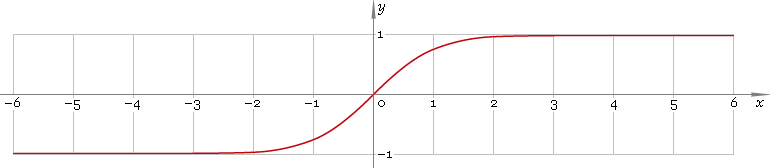The Art of Mathematics

# tanh or th — hyperbolic tangent function

## 1. Definition

Hyperbolic tangent is defined as

tanhx ≡ (ex − ex) /(ex + ex)

## 2. Graph

Hyperbolic tangent is antisymmetric function defined everywhere on real axis. Its graph is depicted below — fig. 1.Fig. 1. Graph of the hyperbolic tangent function y = tanhx.

Function codomain is limited to the range (−1, 1).

## 3. Identities

Base:

tanh2x + sech2x = 1

By definition:

tanhx ≡ sinhx /coshx ≡ 1 /cothx

Property of antisymmetry:

tanh−x = −tanhx

Half-argument:

tanh(x/2) = (coshx − 1) /sinhx
tanh(x/2) = sinhx /(1 + coshx)
tanhx = 2 tanh(x/2) /[1 + tanh2(x/2)]

Double argument:

tanh(2x) = 2 tanhx /(tanh2x + 1)

Triple argument:

tanh(3x) = (tanh3x + 3 tanhx) /(3 tanh2x + 1)

tanh(4x) = (4 tanh3x + 4 tanhx) /(tanh4x + 6 tanh2x + 1)

Power reduction:

tanh2x = (cosh(2x) − 1) /(cosh(2x) + 1)
tanh3x = (sinh(3x) − 3 sinhx) /(cosh(3x) + 3 coshx)
tanh4x = (cosh(4x) − 4 cosh(2x) + 3) /(cosh(4x) + 4 cosh(2x) + 3)
tanh5x = (sinh(5x) − 5 sinh(3x) + 10 sinhx) /(cosh(5x) + 5 cosh(3x) + 10 coshx)

Sum and difference of arguments:

tanh(x + y) = (tanhx + tanhy) /(1 + tanhx tanhy)
tanh(xy) = (tanhx − tanhy) /(1 − tanhx tanhy)

Product:

tanhx tanhy = [cosh(x + y) − cosh(xy)] /[cosh(x + y) + cosh(xy)]

Sum:

tanhx + tanhy = sinh(x + y) /(coshx coshy)
tanhx − tanhy = sinh(xy) /(coshx coshy)

## 4. Derivative and indefinite integral

Hyperbolic tangent derivative:

tanh′x = sech2x ≡ 1 /cosh2x

Indefinite integral of the hyperbolic tangent:

∫ tanhx dx = ln(coshx) + C

where C is an arbitrary constant.

## 5. How to use

To calculate hyperbolic tangent of the number:

``tanh(−1);``

To get hyperbolic tangent of the complex number:

``tanh(−1+i);``

To get hyperbolic tangent of the current result:

``tanh(rslt);``

To get hyperbolic tangent of the angle z in calculator memory:

``tanh(mem[z]);``

## 6. Support

Hyperbolic tangent of the real argument is supported in free version of the Librow calculator.

Hyperbolic tangent of the complex argument is supported in professional version of the Librow calculator.